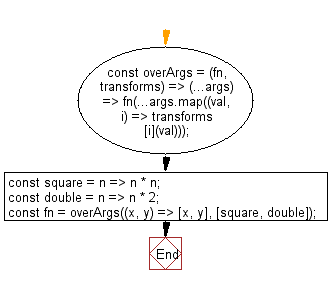# JavaScript : Create a function that invokes the provided function with its arguments transformed

## JavaScript fundamental (ES6 Syntax): Exercise-172 with Solution

Write a JavaScript program to create a function that invokes the provided function with its arguments transformed.

• Use Array.prototype.map() to apply transforms to args in combination with the spread operator (...) to pass the transformed arguments to fn.

Sample Solution:

JavaScript Code:

``````//#Source https://bit.ly/2neWfJ2
const overArgs = (fn, transforms) => (...args) => fn(...args.map((val, i) => transforms[i](val)));
const square = n => n * n;
const double = n => n * 2;
const fn = overArgs((x, y) => [x, y], [square, double]);
console.log(fn(9,3));
console.log(fn(5,2));
```
```

Sample Output:

```[81,6]
[25,4]
```

Pictorial Presentation:Flowchart:Live Demo:

See the Pen javascript-basic-exercise-172-1 by w3resource (@w3resource) on CodePen.

Improve this sample solution and post your code through Disqus

What is the difficulty level of this exercise?

Test your Programming skills with w3resource's quiz.

﻿